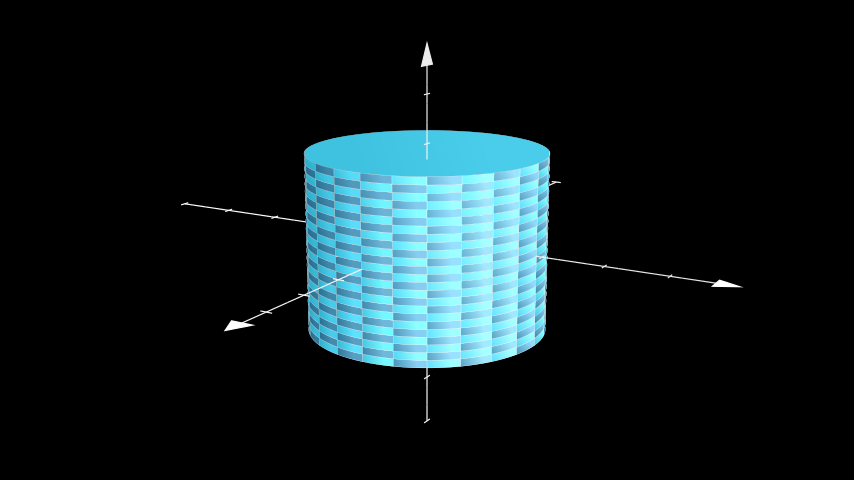# Cylinder#

Qualified name: `manim.mobject.three\_d.three\_dimensions.Cylinder`

class Cylinder(radius=1, height=2, direction=array([0., 0., 1.]), v_range=[0, 6.283185307179586], show_ends=True, resolution=(24, 24), **kwargs)[source]#

Bases: `Surface`

A cylinder, defined by its height, radius and direction,

Parameters

• height (float) – The height of the cylinder.

• direction (np.ndarray) – The direction of the central axis of the cylinder.

• v_range (Sequence[float]) – The height along the height axis (given by direction) to start and end on.

• show_ends (bool) – Whether to show the end caps or not.

• resolution (Sequence[int]) – The number of samples taken of the `Cylinder`. A tuple can be used to define different resolutions for `u` and `v` respectively.

Examples

Example: ExampleCylinder```from manim import *

class ExampleCylinder(ThreeDScene):
def construct(self):
axes = ThreeDAxes()
self.set_camera_orientation(phi=75 * DEGREES, theta=30 * DEGREES)
```

Methods

 `add_bases` Adds the end caps of the cylinder. `func` Converts from cylindrical coordinates to cartesian. `get_direction` Returns the direction of the central axis of the `Cylinder`. `set_direction` Sets the direction of the central axis of the `Cylinder`.

Attributes

 `animate` Used to animate the application of any method of `self`. `animation_overrides` `color` `depth` The depth of the mobject. `fill_color` If there are multiple colors (for gradient) this returns the first one `height` The height of the mobject. `n_points_per_curve` `sheen_factor` `stroke_color` `width` The width of the mobject.

Adds the end caps of the cylinder.

Return type

None

func(u, v)[source]#

Converts from cylindrical coordinates to cartesian.

Parameters
• u (float) – The height.

• v (float) – The azimuthal angle.

Returns

Points defining the `Cylinder`.

Return type

`numpy.ndarray`

get_direction()[source]#

Returns the direction of the central axis of the `Cylinder`.

Returns

direction – The direction of the central axis of the `Cylinder`.

Return type

`numpy.array`

set_direction(direction)[source]#

Sets the direction of the central axis of the `Cylinder`.

Parameters

direction (`numpy.array`) – The direction of the central axis of the `Cylinder`.

Return type

None